# Chapter 6 Regression Trees

Regression trees are an extension of classification trees where the outcome no longer represents categories (e.g., it snowed or did not snow), but instead the outcome is a continuous or interval type. For example, earlier in this book, distributions of college admission rates were explored, this represented a continuous or interval type attribute as the data took on many different values. Regression trees differ from classification trees in that the outcome of the model is a prediction of the continuous quantity rather than predicting if an observation belongs to a specific category. For example, instead of predicting whether it snows or not, a regression tree would predict how much snow fell, such as 5.5 inches. The difference in the predicted value has a few implications for the model, first, the accuracy measure used to evaluate the model needs to differ as it is unlikely that the prediction will be the same for any of the data in a regression tree, secondly, the prediction task is now much more difficult. These two observations need to be kept in mind when working with a regression tree.

Much of the machinery is similar between regression and classification trees, however. Most notably, the same tree type structure will be used to make the prediction. Also, to parallel the classification trees explored so far, the regression trees used in this text will assume that only two splits can occur at any point along the tree. There are also differences between the classification and regression trees. Already discussed is the outcome attribute is continuous for a regression tree, therefore the predicted value will represent one that is continuous as well instead of representing a category. Furthermore, since the predictions are done on a continuous scale, a different measure of overall model fit or accuracy will have to be used. This chapter will explore those details in turn. But first, an example of a regression tree.

## 6.1 Predicting ACT Score

The first example of using a regression tree will attempt to predict the median ACT score for a college institution using other attributes that describe the type of college. Before getting into the model, the packages and loading of the data needs to be done.

The following code will load the packages to use for the fitting of the regression tree and loading of the college scorecard data that was used earlier in the book (see Chapters 2 and 3).

library(tidyverse)
library(ggformula)
library(mosaic)
library(rpart)
library(rsample)
library(rpart.plot)
library(statthink)
library(parttree)

# Set theme for plots
theme_set(theme_statthinking(base_size = 14))

file = "https://raw.githubusercontent.com/lebebr01/statthink/master/data-raw/College-scorecard-clean.csv",
guess_max = 10000
)

### 6.1.2 Visualize distributions

Exploring the distribution of the variable of interest is often the first step in an analysis. A density figure is used to understand

gf_density(~ actcmmid, data = colleges) %>%
gf_labs(x = "Median college ACT score")
## Warning: Removed 730 rows containing non-finite values (stat_density).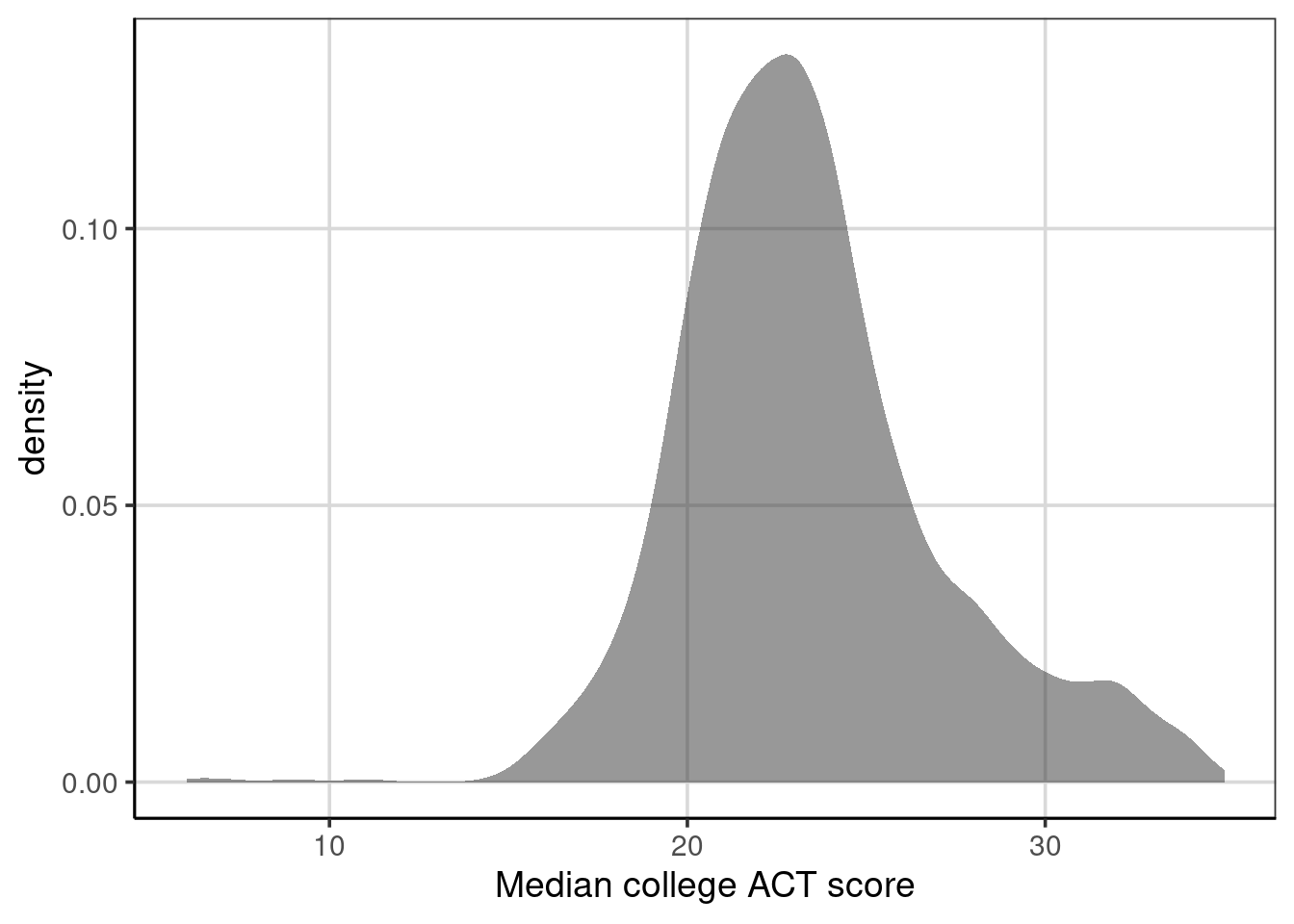Figure 6.1: Density curve of median college ACT score.

1. What are the primary features of the distribution for median ACT score of the college?
2. Could there be concerns about specific features of this distribution if we are looking to do an analysis on this?

## 6.2 Continuous Association

It is often interesting to estimate whether two continuous/interval attributes are associated or related to one another. This can be done with a statistic called the correlation. The correlation measures the degree to which the two attributes move together or not. For example, if one attribute increases does it give information about whether the second attribute increases (ie., a positive correlation) or decreases (ie., a negative correlation)? Before getting to the computation, exploring a sample can be helpful.

First, to get a sense to what the correlation represents, it is useful to explore a scatterplot. The scatterplot depicts two attributes and places a point where the two attributes intersect. For this first example, a scatterplot with median college ACT score and admission rate are explored.

gf_point(actcmmid ~ adm_rate, data = colleges, size = 3) %>%
y = 'Median College ACT Score')
## Warning: Removed 730 rows containing missing values (geom_point).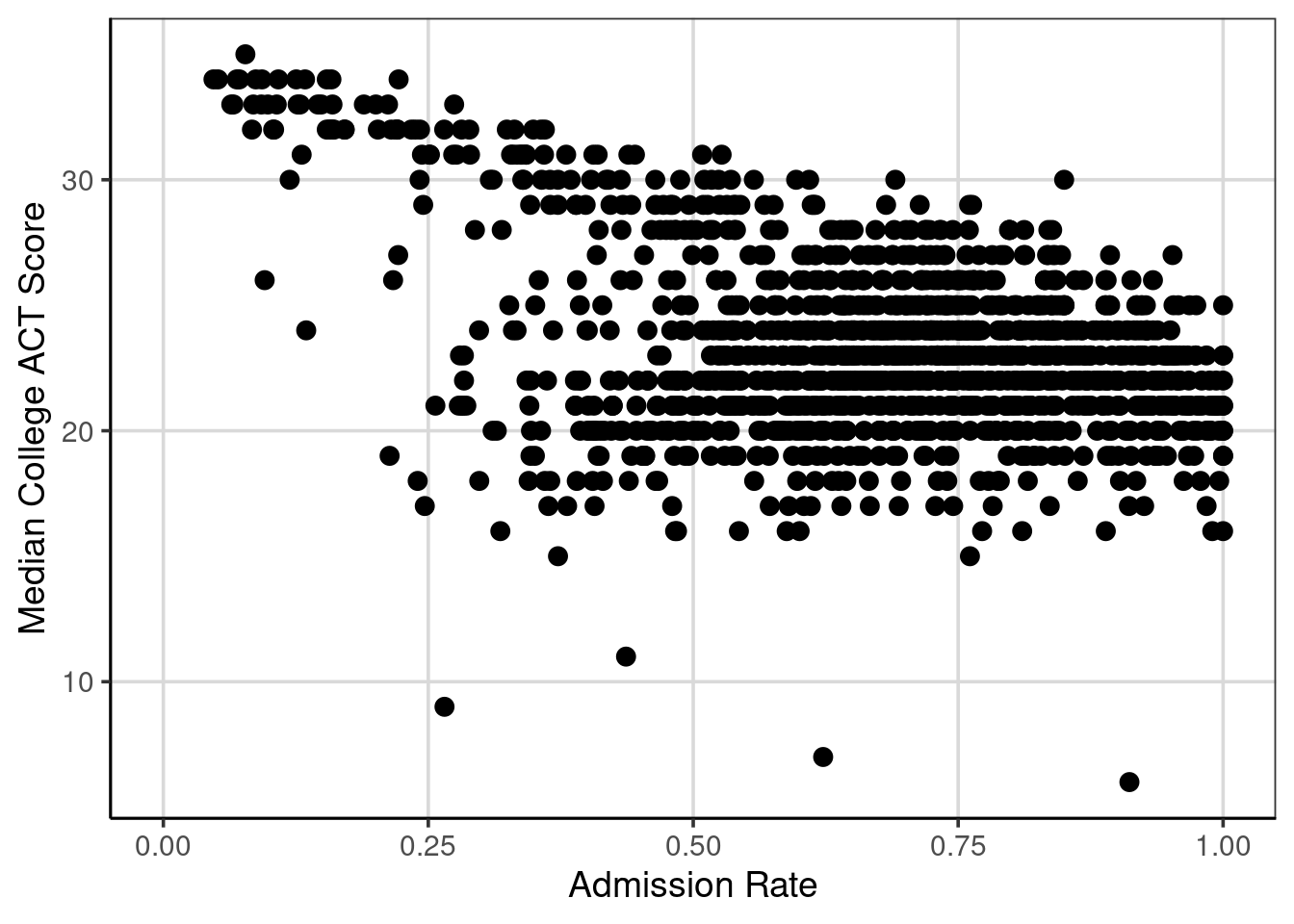Figure 6.2: Scatterplot of median college ACT score by college admission rate.

Figure 6.2 shows the bivariate relationship between median college ACT score and the college admission rate. If these two attributes are unrelated, it would not be possible to identify a trend in the scatterplot. However, in Figure 6.2, there is a noticeable trend. As median college ACT scores increase, the admission rates tend to decrease. This is an example of a negative trend and would represent a negative relationship, association, or correlation.

Although there is a trend in the relationship, there are exceptions to this rule. For instance, notice the points that have very low median college ACT scores, there are cases that have lower admission rates (about 0.25 or 25%) and some have higher admission rates (above 0.5 or 50%). Focusing on the single point at about 0.25 admission rate and 9 median college ACT score, most of the median college ACT scores for an admission rate around 0.25 are above 30, with a few clustered around 20. This indicates the variation and imperfect relationship between the two attributes. The mantra, “correlation does not mean causation” is common in statistics courses and this variation and imperfect relationship shows one example of this. In short, it requires very specific designs to the data collection to be able to make very strong statements about causation, most often the data available represent observations that were simply observed rather than being part of a more stringent experimental design.

The correlation statistic provides a numeric summary of the degree of relationship between two quantitative attributes. These can be estimated with the cor() function. The primary argument is a formula like has been used before and is similar to the gf_point() function used above. The data argument is specified and one other argument may be helpful when there are data missing. This is the use = 'complete.obs' argument. Specifying this argument with 'complete.obs' tells the cor() function to remove any missing data on the two attributes being specified and keep any data where pairs of data are present.

cor(actcmmid ~ adm_rate, data = colleges, use = 'complete.obs')
##  -0.4058855

The correlation estimate depicted here is -0.41, which carries two pieces of information. One, it shows the direction of association, here negative means that as one attribute increases the other tends to decrease (an inverse relationship). This was what was noticed in 6.2. The second element the correlation shows is the magnitude of association. The correlation is standardized, meaning that regardless of the attributes used for the computation, the correlation will always be in the same range. The correlation will exclusively be between -1 to +1. Values of -1 or +1 indicate perfect negative or positive relationships respectively. A correlation of 0 means no relationship. The example above had a correlation of -0.41, which means the relationship is negative/inverse (as one attribute increases, the other tends to decrease) and the magnitude of association is about in between -1 and 0, but slightly closer to 0. What is considered a large, medium, or small association is largely dependent on the industry, but with education or social science data, this type of association would likely be considered a moderate or medium degree of association.

The correlation makes one other assumption about the data. The correlation computed above and considered in this text is sometimes referred to as the Pearson correlation. This correlation assumes the data are best represented by a straight line or a linear relationship. If the relationship is not linear, but is best represented by a curved line, the correlation used in this course would likely underrepresent the degree of association.

Note: Add some more detail on + or close to 0 correlations.

### 6.2.1 Correlation Computation

The correlation can be represented by the following formula. In this formula, $$\bar{x}, \bar{y}$$ are the means for x and y respectively. THe terms, $$x_{i}$$, and $$y_{i}$$, represent the individual data elements for each attribute. The numerator of the formula represents the deviations from the mean for the two attributes, which are multiplied together and added. The numerator is also referred to as the covariance and measures the degree to which the two attributes move together.

$r = \frac{\sum (x_{i} - \bar{x})(y_{i} - \bar{y})}{\sqrt{\sum(x_{i} - \bar{x})^2}\sqrt{\sum(y_{i} - \bar{y})^2}}$

The denominator of the correlation are the standard deviation of the two attributes. These act to standardize the correlation so that no matter which attributes are entered into the formula, the correlation will always be between -1 and +1.

## 6.3 First Regression Tree

Another, and related to the correlation, way to explore the relationship between two quantitative attributes is through the fitting a regression tree. A regression tree is similar to a classification tree, however now the output is a numeric or continuous type variable that takes on many different values. In the classification tree example, the focus in this class was predicting if a case belonged to one of two classes. The regression tree, in contrast, will predict the numeric variable with many potential values rather than just two. This will have implications for how the model is evaluated for accuracy as well as how well the model does at predicting specific values. These will be discussed in more detail later.

The syntax for fitting a regression tree is very similar in R compared to the classification tree. The same function, rpart() is used and the function rpart.plot() will be used to visualize the fitted regression tree similar to before. The primary argument to the rpart() function is a formula where the left-hand side is the attribute of interest and the right hand side contains attributes that help predict the outcome. In the example below, the median college ACT score (actcmmid) is the outcome attribute and the college admission rate (adm_rate) is used as the sole continuous attribute used to predict the median college ACT score. The data argument is also specified and the only difference here between a classification tree and the regression tree here is the method argument. In the regression tree the method argument should be set to method = 'anova'. This tells the rpart() function that the outcome is numeric and that an anova method should be used in the model fitting. The anova stands for Analysis of Variance and we will discuss this in more detail moving forward.

act_reg <- rpart(actcmmid ~ adm_rate, data = colleges,
method = "anova")

rpart.plot(act_reg, roundint = FALSE, type = 3, branch = .3)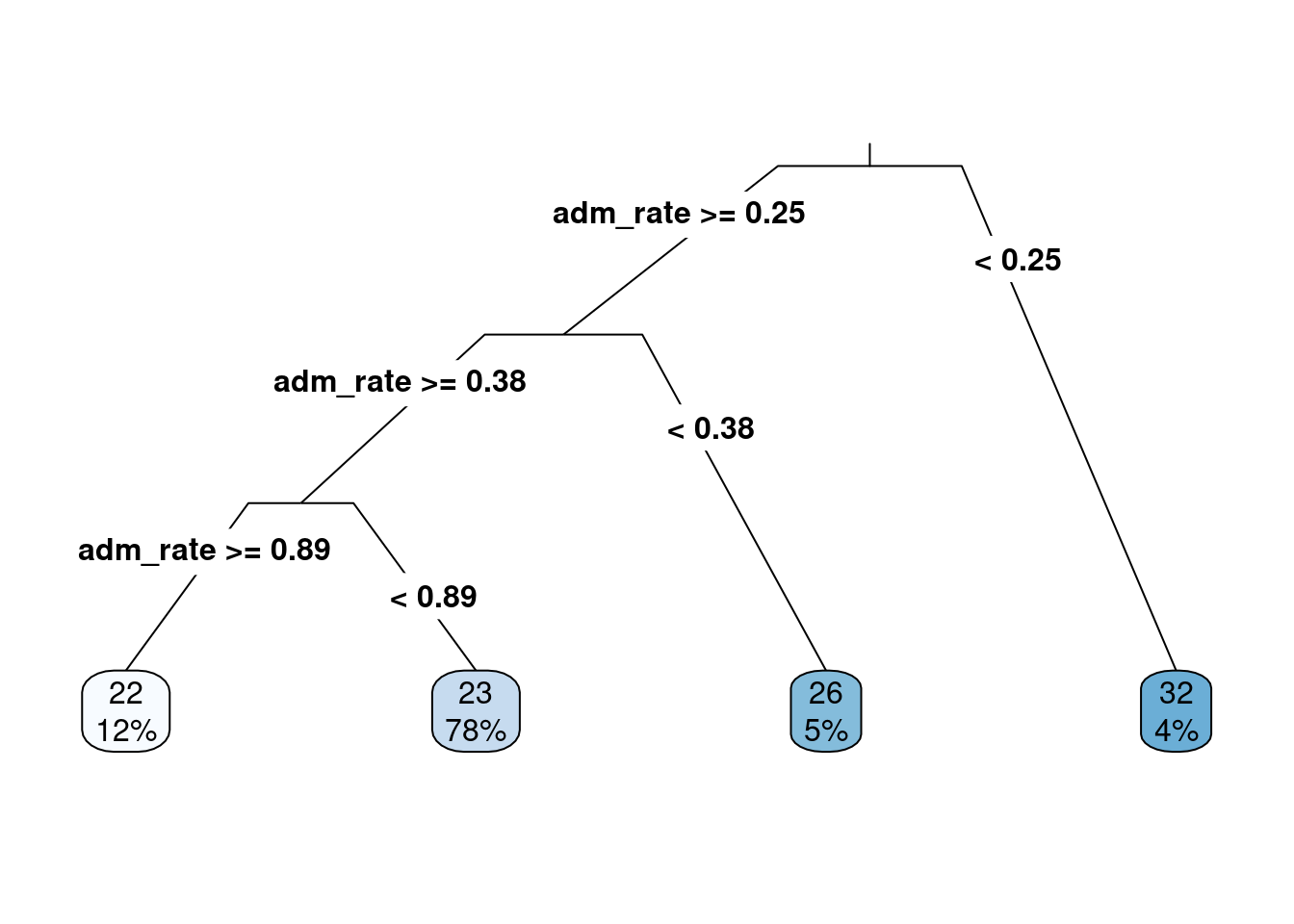Figure 6.3: The first regression tree predicting median college ACT score based on the admission rate.

The output from the regression tree is similar to that from a classification tree. One major difference however is that the predicted values in the end are numeric quantities instead of classes and the probabilities that were shown previously are not shown here as there is not a probability of being in a class. The percentage of cases in the predicted nodes at the end of the tree are still shown. The logic for following the tree is the same as before where each split can have two new paths to follow and then the variable(s) are re-evaluated after the first split to see if additional splits can help predict the outcome of interest.

Below is a figure that builds on the scatterplot we saw above. Vertical lines are shown that indicate the splits that were established from the regression tree in Figure 6.3. These splits are where the end buckets lie and all of the data points residing in a single area have the same median ACT score. For example, all schools in the left-most quadrant of Figure 6.4 would all have the same predicted median ACT score of 32. In reality, one can see that the value of 32 is correct for some of the schools, but there are others in this quadrant that have median ACT scores of less than 20. The next section will explore how accuracy for the regression tree can be evaluated.

gf_point(actcmmid ~ adm_rate, data = colleges, color = 'gray55') %>%
gf_labs(y = "Median college ACT score",
title = "Log salary by number of home runs") +
geom_parttree(data = act_reg, aes(fill = actcmmid), alpha = 0.3) +
scale_fill_continuous("ACT")
## Warning: Removed 730 rows containing missing values (geom_point).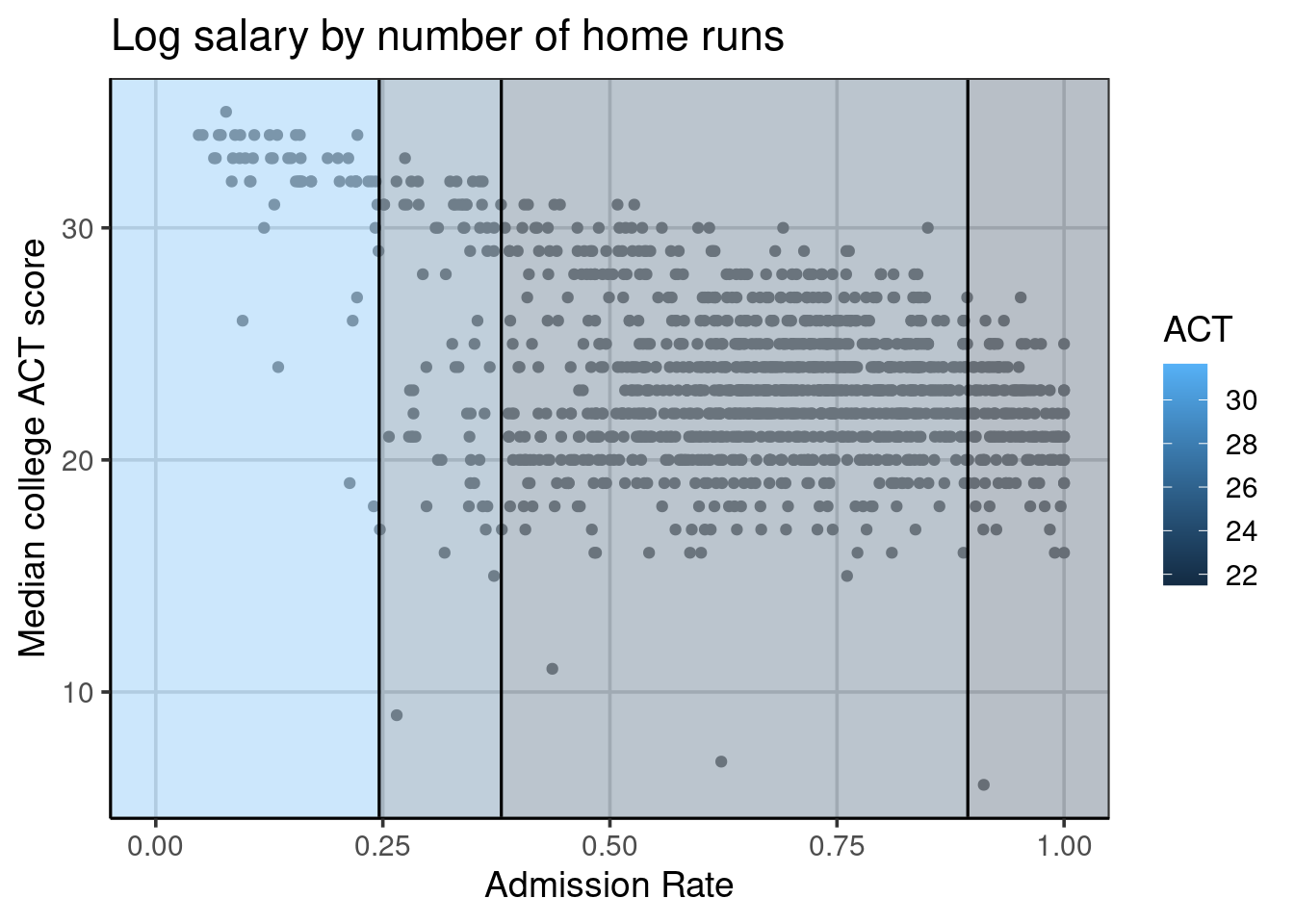Figure 6.4: Visual depiction of the regression tree splits.

## 6.4 Evaluating accuracy

In the classification tree example, a natural metric to evaluate how well the model was doing was the classification accuracy. Classification accuracy was often best computed for each class instead of a single overall accuracy statistic. In the regression tree example, there is no class membership, instead the original observed college ACT score and the predicted college ACT scores can be compared. One measure that could be used for accuracy is on average how far do the predicted scores deviate from the observed scores. Mathematically, this would look like:

$accuracy = predicted - observed$

However, this will have issues, as when the deviation was computed, those values above and below will offset one another. Therefore, simply computing the accuracy as the predicted score minus the observed score will on average be 0. Similarly to the standard deviation, we could square the difference between the predicted and observed values to get the mean square error. We could also take the square root of the mean square error (MSE) to get the root mean square error (RMSE), the benefit of the RMSE over the MSE is that the RMSE will be on the same metric as the outcome of interest. Mathematically, these can be represented as:

$MSE = \frac{\sum (predicted - observed) ^ 2}{n}$

$RMSE = \sqrt{\frac{\sum (predicted - observed) ^ 2}{n}}$

Another statistic can also be useful here, the mean absolute error (MAE). This statistic was first introduced in chapter 3 and is similar to the RMSE above, but except for squaring values and taking the square root, the absolute value is used instead. This has the strength of never squaring values, so the metric is the same as the outcome, but has the weakness of having weaker statistical properties. The mathematical computation can be shown as:

$MAE = \frac{\sum \left|predicted - observed \right| }{n}$

The below code chunk computes the deviation labeled as error. The first 10 rows are shown along with the institution name (instnm), median ACT score (actcmmid), and the predicted median ACT score (act_pred). Notice within the error column that there are some values above and below zero. You can see the values that have a negative error have an actual median ACT score less than the predicted values. Conversely, those that have positive errors have actual median ACT scores greater than the predicted ACT value.

colleges_pred <- colleges %>%
drop_na(actcmmid) %>%
mutate(act_pred = predict(act_reg),
error = actcmmid - act_pred) %>%
select(instnm, actcmmid, act_pred, error)
head(colleges_pred, n = 10)
## # A tibble: 10 × 4
##    instnm                              actcmmid act_pred error
##    <chr>                                  <dbl>    <dbl> <dbl>
##  1 Alabama A & M University                  18     21.5 -3.50
##  2 University of Alabama at Birmingham       25     21.5  3.50
##  3 University of Alabama in Huntsville       28     23.1  4.89
##  4 Alabama State University                  18     21.5 -3.50
##  5 The University of Alabama                 28     23.1  4.89
##  6 Auburn University at Montgomery           22     23.1 -1.11
##  7 Auburn University                         27     23.1  3.89
##  8 Birmingham Southern College               26     23.1  2.89
##  9 Faulkner University                       20     23.1 -3.11
## 10 Huntingdon College                        22     23.1 -1.11

The df_stats() function can used to compute summary statistics for the error attribute which represented the difference between the observed and predicted college ACT score. A variety of statistics are computed on the error attribute including the mean, median, variance (var), standard deviation (sd), minimum (min), and maximum (max).

colleges_pred %>%
df_stats(~ error, mean, median, var, sd, min, max)
##   response          mean    median      var       sd       min      max
## 1    error -5.043527e-16 -0.111001 9.919378 3.149504 -16.68116 7.888999

Notice from the statistics computed, the mean and median of the deviation or raw error (i.e., $$predicted - observed$$), the values are very close to zero and are practically zero for the mean. This is occurring due to the positive and negative errors are offsetting one another. The median isn’t exactly zero as the distribution of errors are not perfectly symmetric. Figure 6.5 shows the distribution of errors. Notice there is a longer tail on the negative side, meaning that there are some predicted values that are much larger than the observed median ACT score. This is also shown by the min and max statistics computed above.

gf_density(~ error, data = colleges_pred) %>%
gf_labs(x = "Error = Predicted - Observed")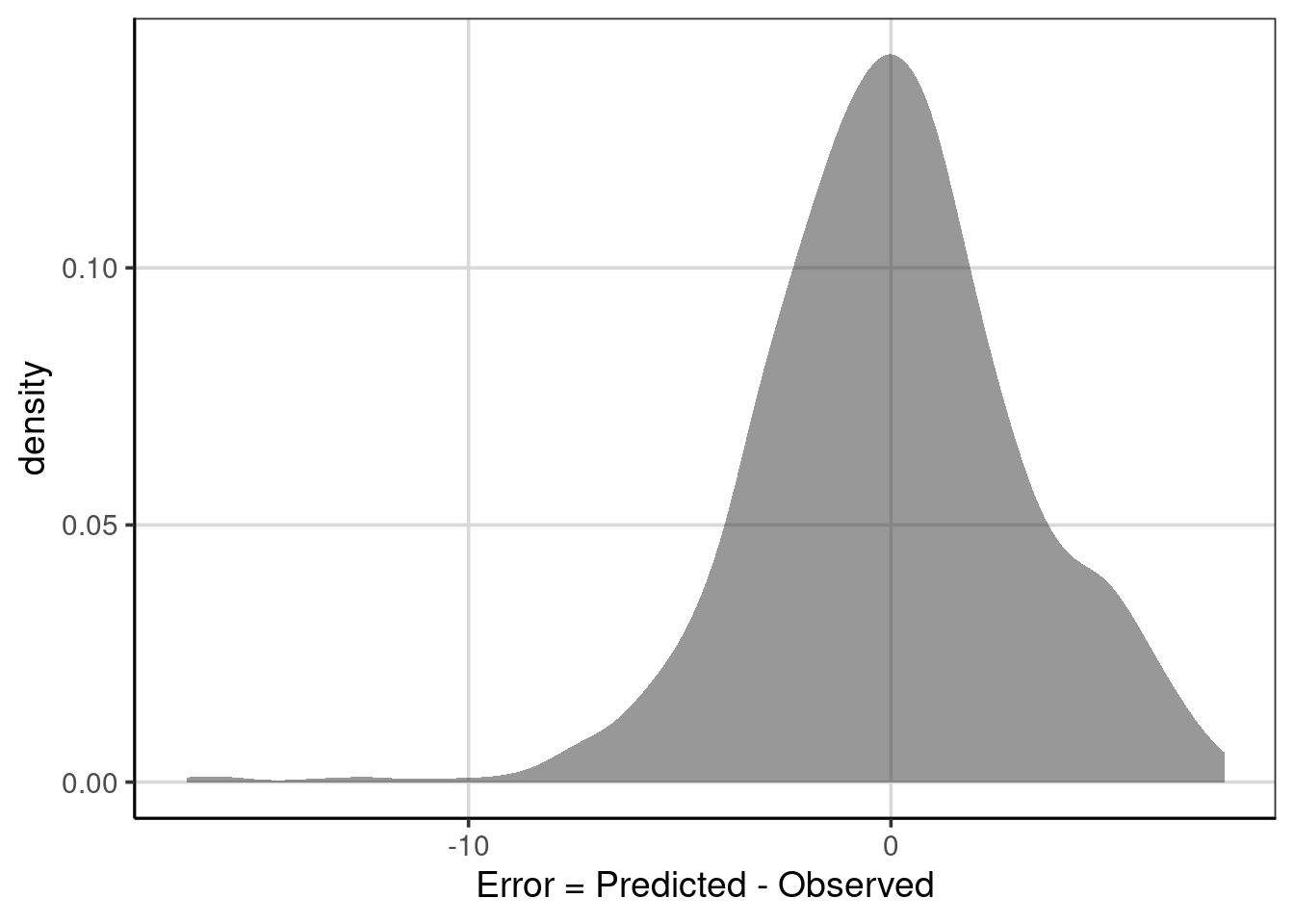Figure 6.5: Distribution of error deviations from the regression tree model.

Circling back to the final statistic computed above, the variance (var) and standard deviation (sd) of the error term represents the MSE and RMSE respectively and are interpretable. In general, larger values of these statistics indicate less accuracy, that is, on average the squared deviations of the predicted minus the observed are larger. In practice, the RMSE is often used more than the MSE as the metric is the same as the outcome, in this case the median ACT scores. The RMSE of 3.15 says that on average the predicted median institution ACT scores are about 3.15 away from the observed median institution ACT score.

The mean absolute error can be computed similarly, except instead of using the raw deviations (ie., errors), the absolute value of the deviations needs to be computed first. This is done using the abs() function shown below and specified as abs(error). The error term represents the errors computed as the difference in the predicted and observed median ACT scores. Similar to the statistics computed above, the mean, median, minimum, and maximum statistics are computed.

colleges_pred %>%
df_stats(~ abs(error), mean, median, min, max)
##     response     mean   median      min      max
## 1 abs(error) 2.396454 1.888999 0.111001 16.68116

When interpreting these statistics, the mean and median column would represent the mean and median absolute error and are on the same scale as the outcome attribute, in this case the median ACT score. Therefore, the value of 2.4 states that on average the predicted median ACT scores are within 2.4 from the observed scores. The median is interpreted similarly, but instead of being the average absolute deviation, this represents the median absolute deviation. Finally, notice how the absolute value worked, the minimum statistic is positive, whereas before it was negative. The largest negative deviation/error from before is now the largest shown in the maximum column. The absolute value of the errors can be shown graphically to fully understand the new distribution.

gf_density(~ abs(error), data = colleges_pred) %>%
gf_labs(x = "Error = |Predicted - Observed|")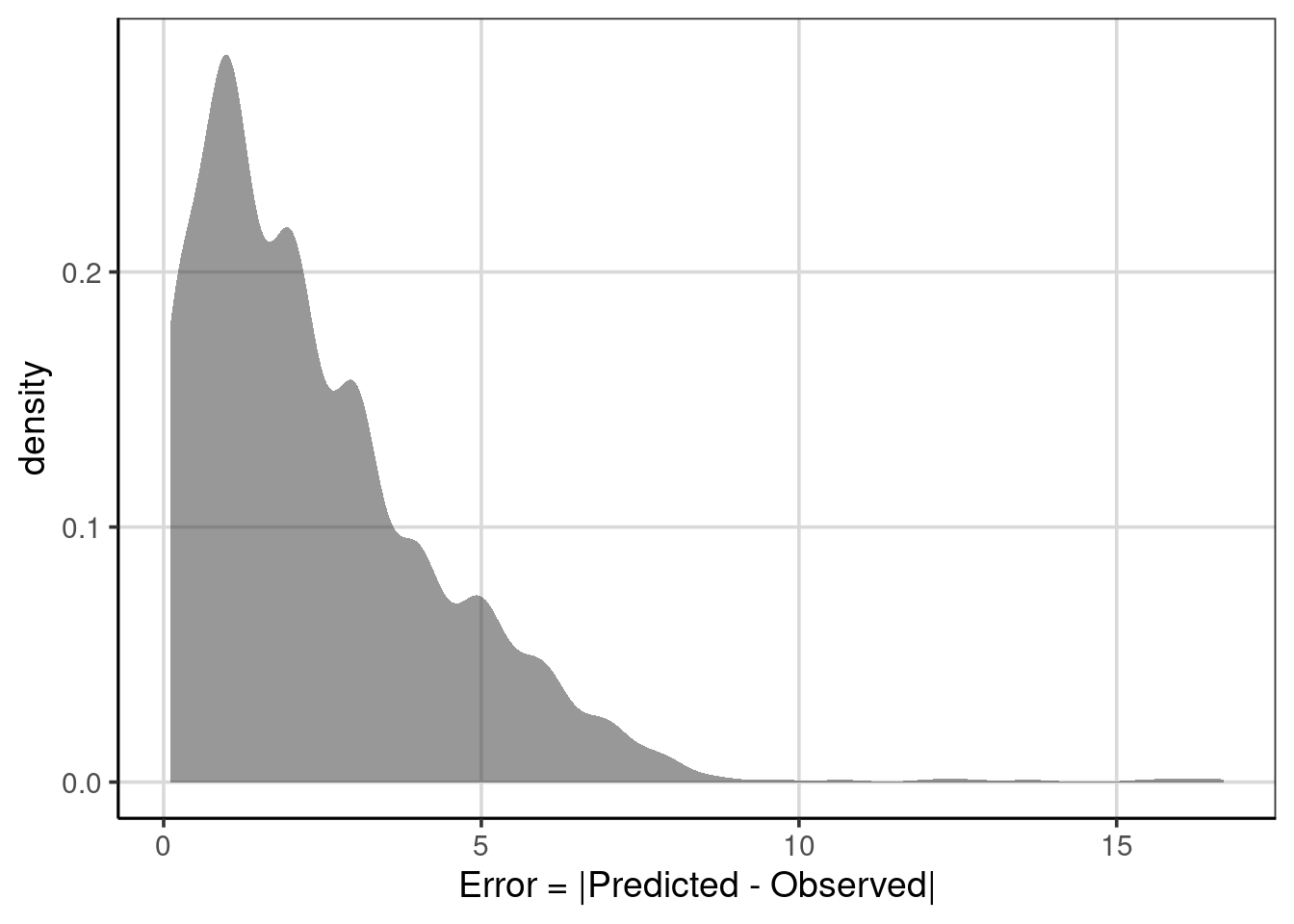Figure 6.6: The distribution of errors after taking the absolute value .

This distribution is not symmetric, rather it is more like an exponential distribution with many values close to zero and rather quickly decreasing in the number of errors that are larger. This indicates that much of the predictions are close to the observed value with a few that are further away indicating worse performance.

It is also worth mentioning that these accuracy statistics are scale dependent. Therefore if the scales of two outcomes are different, the MAE, MSE, or RMSE are not directly comparable without standardization. For example, if instead of median ACT scores, the outcome was median SAT scores, the accuracy statistics would naturally be larger due to SAT scores being larger. SAT scores for individual sections range from 200 to 800 instead of ACT scores ranging from 0 to 36. The MAE, MSE, or RMSE statistics would look larger due to scale differences, but that would not necessarily mean the model is doing worse. To compare these two outcomes, the outcomes or the accuracy statistics would need to be put on the same scale.

### 6.4.1 Conditional Error

Similar to problems with just using the overall classification accuracy with classification trees, it can be helpful to use conditional error with regression trees. The conditional error can help to identify areas of the model that are performing worse, more specifically, to understand where the model has larger errors. To do this with some descriptive statistics, the df_stats() function can be used where the formula input can include two attributes, the error on the left side of the ~ and the attribute to explore the conditional error by on the right hand side. The formula used below explores the MAE across different median ACT scores using the formula, abs(error) ~ actcmmid. The function length is used to calculate how many schools are in each of the computations.

colleges_pred %>%
df_stats(abs(error) ~ actcmmid, mean, median, min, max, length)
##      response actcmmid       mean     median        min       max length
## 1  abs(error)        6 15.5032258 15.5032258 15.5032258 15.503226      1
## 2  abs(error)        7 16.1110010 16.1110010 16.1110010 16.111001      1
## 3  abs(error)        9 16.6811594 16.6811594 16.6811594 16.681159      1
## 4  abs(error)       11 12.1110010 12.1110010 12.1110010 12.111001      1
## 5  abs(error)       15  9.3960802  9.3960802  8.1110010 10.681159      2
## 6  abs(error)       16  7.0523290  7.1110010  5.5032258  9.681159     11
## 7  abs(error)       17  6.1276847  6.1110010  4.5032258  8.681159     19
## 8  abs(error)       18  5.4476764  5.1110010  3.5032258 13.642857     32
## 9  abs(error)       19  4.0157276  4.1110010  2.5032258 12.642857     59
## 10 abs(error)       20  2.9102946  3.1110010  1.5032258  5.681159    117
## 11 abs(error)       21  1.8594407  2.1110010  0.5032258  4.681159    156
## 12 abs(error)       22  1.0878288  1.1110010  0.4967742  3.681159    166
## 13 abs(error)       23  0.3555768  0.1110010  0.1110010  2.681159    174
## 14 abs(error)       24  1.0982335  0.8889990  0.8889990  7.642857    155
## 15 abs(error)       25  2.0242671  1.8889990  0.6811594  3.496774    101
## 16 abs(error)       26  2.9769006  2.8889990  0.3188406  5.642857     70
## 17 abs(error)       27  3.9392465  3.8889990  3.8889990  5.496774     47
## 18 abs(error)       28  4.7721736  4.8889990  2.3188406  4.888999     44
## 19 abs(error)       29  5.5355597  5.8889990  2.6428571  5.888999     31
## 20 abs(error)       30  5.7496633  6.8889990  1.6428571  6.888999     25
## 21 abs(error)       31  5.5947035  5.3188406  0.6428571  7.888999     22
## 22 abs(error)       32  2.1915114  0.3571429  0.3571429  6.318841     26
## 23 abs(error)       33  1.7545894  1.3571429  1.3571429  7.318841     15
## 24 abs(error)       34  2.3571429  2.3571429  2.3571429  2.357143     12
## 25 abs(error)       35  3.3571429  3.3571429  3.3571429  3.357143      1

Notice that there is a row for each median ACT score found in the data and that each statistic is computed for each unique median ACT score. Procedurally, the data are split into compartments and the statistics are computed on the data that fit into those compartments. The MAE (shown by the mean column) shows larger errors at low values of median ACT score, which decreases quickly until a median ACT score of 23, then increases slightly, then decreases again for higher values.

Before spending too much time with this table, take a peak at the last column, labeled length. This column tells how many data points are in each of the compartments to do the calculation. In general, compartments with less data in them will produce statistics that are more unstable. For instance, there are very few schools with very low ACT scores, for example, scores of 15 and under. Furthermore, there is only 1 school with a median ACT score of 35. When there are small numbers for some of these categories, it can be helpful to combine them for evaluation of model accuracy. In this example, I’m going to collapse scores less than 15 into one category and 34 and higher into another. The other median ACT scores I’m going to leave by themselves.

colleges_pred <- colleges_pred %>%
mutate(act_recode = ifelse(actcmmid <= 15, '15 or smaller',
ifelse(actcmmid >= 34, '34 or larger', actcmmid)))

The mutate() function above is used to collapse the categories and create a new attribute named act_recode. Then, this new attribute is used to compute the MAE and other statistics to evaluate conditional model performance. Notice that the length column at the end no longer has categories with a single data point in them, rather the smallest is now 6, which is still small, but at least there are more than one data point in them. Similar trends to those discussed above pertain here.

conditional_error <- colleges_pred %>%
df_stats(abs(error) ~ act_recode, mean, median, min, max, length)

conditional_error
##      response    act_recode       mean     median       min       max length
## 1  abs(error) 15 or smaller 13.1997579 13.8071134 8.1110010 16.681159      6
## 2  abs(error)            16  7.0523290  7.1110010 5.5032258  9.681159     11
## 3  abs(error)            17  6.1276847  6.1110010 4.5032258  8.681159     19
## 4  abs(error)            18  5.4476764  5.1110010 3.5032258 13.642857     32
## 5  abs(error)            19  4.0157276  4.1110010 2.5032258 12.642857     59
## 6  abs(error)            20  2.9102946  3.1110010 1.5032258  5.681159    117
## 7  abs(error)            21  1.8594407  2.1110010 0.5032258  4.681159    156
## 8  abs(error)            22  1.0878288  1.1110010 0.4967742  3.681159    166
## 9  abs(error)            23  0.3555768  0.1110010 0.1110010  2.681159    174
## 10 abs(error)            24  1.0982335  0.8889990 0.8889990  7.642857    155
## 11 abs(error)            25  2.0242671  1.8889990 0.6811594  3.496774    101
## 12 abs(error)            26  2.9769006  2.8889990 0.3188406  5.642857     70
## 13 abs(error)            27  3.9392465  3.8889990 3.8889990  5.496774     47
## 14 abs(error)            28  4.7721736  4.8889990 2.3188406  4.888999     44
## 15 abs(error)            29  5.5355597  5.8889990 2.6428571  5.888999     31
## 16 abs(error)            30  5.7496633  6.8889990 1.6428571  6.888999     25
## 17 abs(error)            31  5.5947035  5.3188406 0.6428571  7.888999     22
## 18 abs(error)            32  2.1915114  0.3571429 0.3571429  6.318841     26
## 19 abs(error)            33  1.7545894  1.3571429 1.3571429  7.318841     15
## 20 abs(error)  34 or larger  2.4340659  2.3571429 2.3571429  3.357143     13

It can be helpful to visualize some of these statistics to help facilitate any trend in the model performance across the median ACT values. A pointrange plot is used to show the average mean absolute error and also the minimum and maximum absolute error values for each median ACT score, using the gf_pointrangeh() function. The formula input for this function takes the following form, y-scores ~ mean-value + min-value + max-value.

gf_pointrangeh(act_recode ~ mean + min + max,
data = conditional_error) %>%
gf_labs(y = "Median ACT Score",
x = "Absolute Error")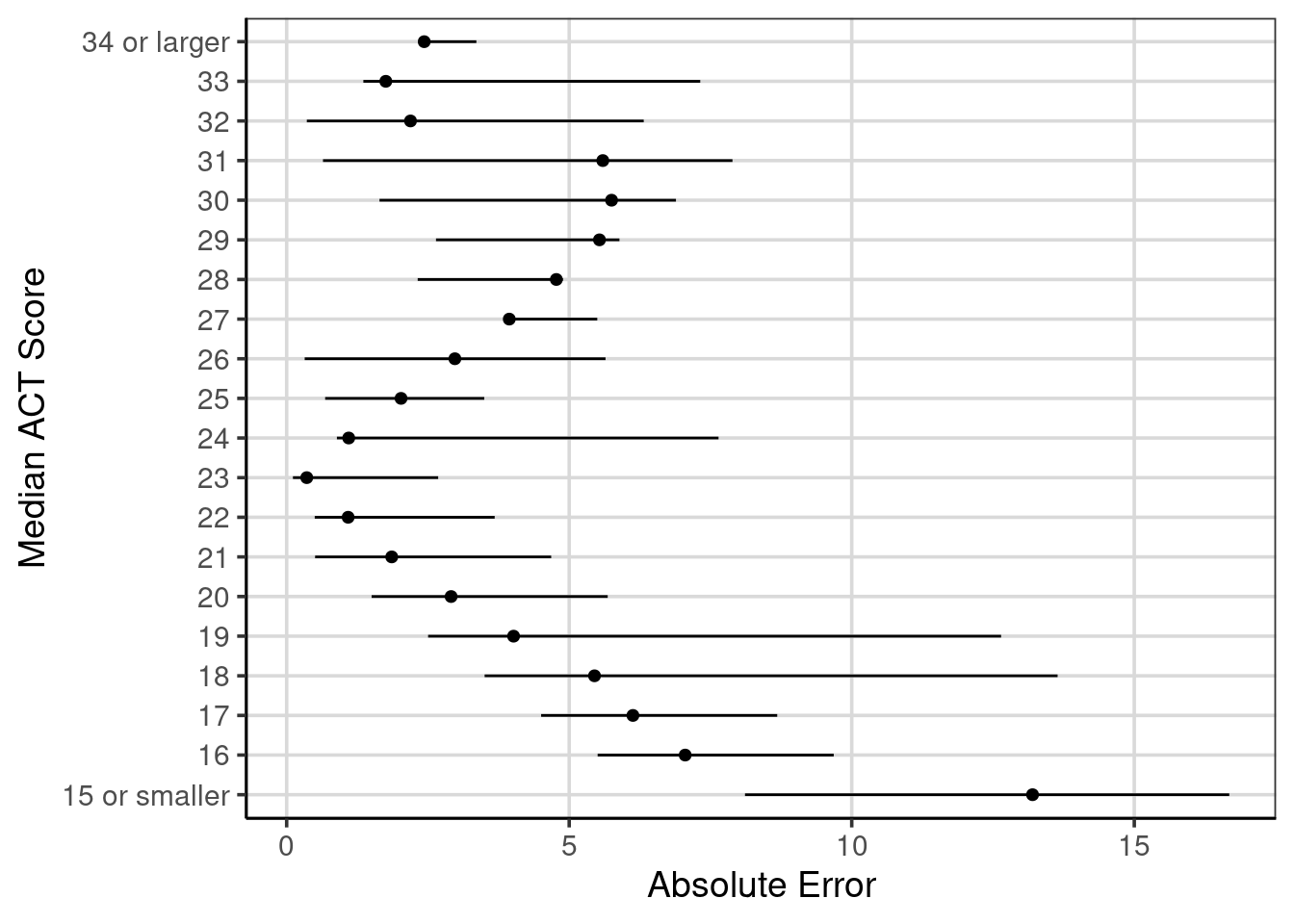Figure 6.7: Ranges of absolute errors across different median ACT scores with mean absolute error.

Figure 6.7 shows these values with the MAE shown with the circles and the horizontal lines extend to the minimum and maximum absolute values for each median ACT score. The trend of decreasing absolute error from small median ACT scores up to a score of 23 is shown. Then the error tends to increase, then decrease again for higher median ACT scores. This shows that the regression tree model is doing well for median ACT scores around 23 and also for median ACT scores around 33.

Another notable feature this figure helps to articulate is the range of absolute errors, for instance, with median ACT scores of 18 or 19, the range of absolute errors range from less than 5 to about 13 or 14. These large range of errors are not as surprising here as the regression tree used to generate these errors is only using a single attribute to help predict median ACT score for institutions, the admission rate. It would make sense that other attributes may help to reduce the error and increase the utility of the model, but this is a good baseline to compare how well other models perform.

#### 6.4.1.1 Explore another attribute

Let’s explore another attribute, the undergraduate enrollment of institutions to see if it is related to the median ACT score. First a scatterplot is shown then the correlation is computed.

gf_point(actcmmid ~ ugds, data = colleges, size = 3) %>%
y = "Median ACT Score")
## Warning: Removed 730 rows containing missing values (geom_point).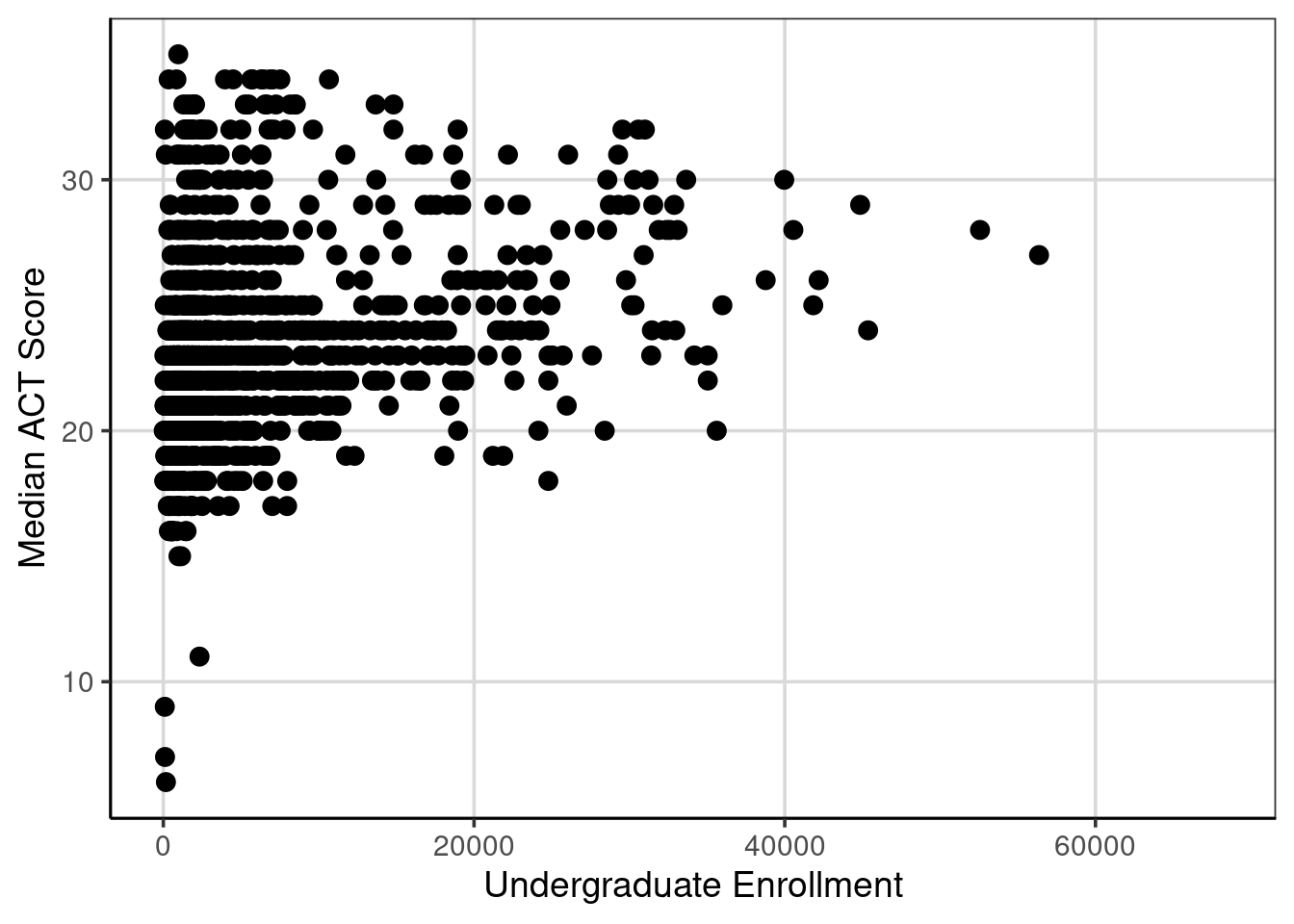The correlation is 0.27 between median ACT score and the undergraduate enrollment of institutions.

act_reg2 <- rpart(actcmmid ~ adm_rate + ugds, data = colleges, method = "anova")

rpart.plot(act_reg2, roundint = FALSE, type = 3, branch = .3)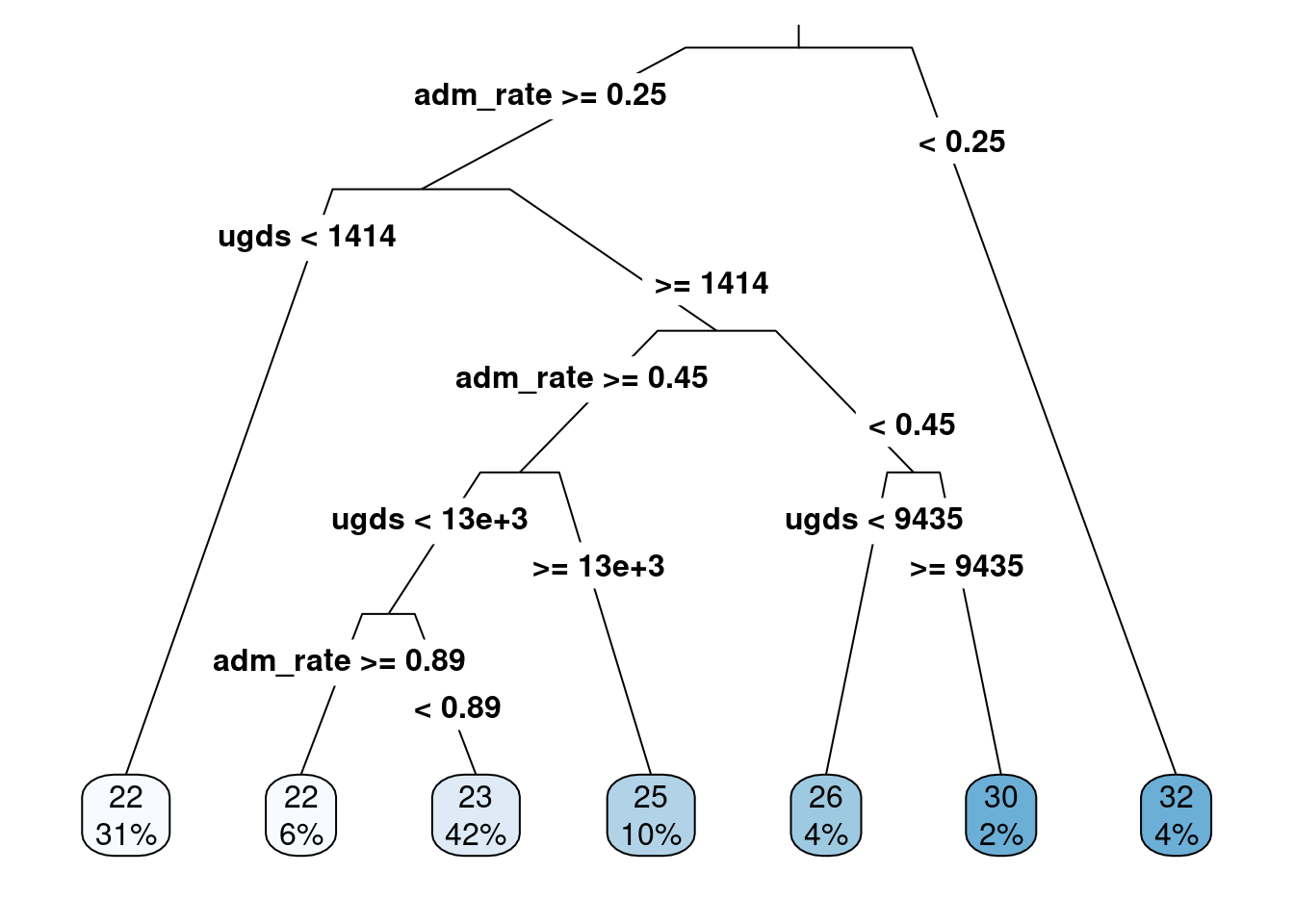Figure 6.8: Regression tree with admission rates and ungraduate enrollments predicting median ACT score

Figure 6.8 shows the regression tree when adding in the undergraduate enrollment attribute in addition to the admission rate. The regression tree shows that the admission rate is the most important attribute in predicting median ACT score (it is the first split in the regression tree), however undergraduate enrollment is important as this is the second split for those with admission rates greater than 25%.

To fully understand how much better this model is from the one with only admission rates included, we can use MAE to evaluate the prediction accuracy. First, the predicted values from the new model and the new errors (predicted - observed) need to be added to the data.

colleges_pred <- colleges_pred %>%
mutate(act_pred2 = predict(act_reg2),
error2 = actcmmid - act_pred2)

colleges_pred
## # A tibble: 1,289 × 7
##    instnm                    actcmmid act_pred error act_recode act_pred2 error2
##    <chr>                        <dbl>    <dbl> <dbl> <chr>          <dbl>  <dbl>
##  1 Alabama A & M University        18     21.5 -3.50 18              21.6 -3.55
##  2 University of Alabama at…       25     21.5  3.50 25              24.8  0.222
##  3 University of Alabama in…       28     23.1  4.89 28              23.3  4.69
##  4 Alabama State University        18     21.5 -3.50 18              21.6 -3.55
##  5 The University of Alabama       28     23.1  4.89 28              24.8  3.22
##  6 Auburn University at Mon…       22     23.1 -1.11 22              23.3 -1.31
##  7 Auburn University               27     23.1  3.89 27              24.8  2.22
##  8 Birmingham Southern Coll…       26     23.1  2.89 26              21.7  4.34
##  9 Faulkner University             20     23.1 -3.11 20              23.3 -3.31
## 10 Huntingdon College              22     23.1 -1.11 22              21.7  0.342
## # … with 1,279 more rows
conditional_error2 <- colleges_pred %>%
df_stats(abs(error2) ~ act_recode, mean, median, min, max, length)

conditional_error2
##       response    act_recode       mean     median       min       max length
## 1  abs(error2) 15 or smaller 11.9076028 13.6583541 6.6583541 15.658354      6
## 2  abs(error2)            16  5.9580028  5.6583541 5.6583541  7.306422     11
## 3  abs(error2)            17  5.4041307  4.6583541 4.5512821  9.153846     19
## 4  abs(error2)            18  4.9394393  3.6583541 3.5512821 13.642857     32
## 5  abs(error2)            19  3.6881805  2.6583541 2.5512821 12.642857     59
## 6  abs(error2)            20  2.3972274  1.6583541 1.5512821  6.153846    117
## 7  abs(error2)            21  1.4072426  0.6583541 0.5512821  5.153846    156
## 8  abs(error2)            22  1.0661678  1.3064220 0.3416459  4.153846    166
## 9  abs(error2)            23  0.8835657  0.3064220 0.3064220  6.590909    174
## 10 abs(error2)            24  1.2956011  0.6935780 0.6935780  7.642857    155
## 11 abs(error2)            25  1.7661025  1.6935780 0.2222222  3.448718    101
## 12 abs(error2)            26  2.6643144  2.6935780 0.1538462  5.642857     70
## 13 abs(error2)            27  3.6386582  3.6935780 2.2222222  5.341646     47
## 14 abs(error2)            28  4.3625148  4.6935780 1.8461538  6.341646     44
## 15 abs(error2)            29  4.0390696  4.2222222 0.5909091  7.341646     31
## 16 abs(error2)            30  4.0587815  3.8461538 0.4090909  6.693578     25
## 17 abs(error2)            31  4.4734457  4.8461538 0.6428571  9.341646     22
## 18 abs(error2)            32  1.9545834  0.3571429 0.3571429 10.341646     26
## 19 abs(error2)            33  1.4939394  1.3571429 1.3571429  3.409091     15
## 20 abs(error2)  34 or larger  2.4340659  2.3571429 2.3571429  3.357143     13
bind_rows(
mutate(conditional_error, model = 'model1'),
mutate(conditional_error2, model = 'model2')
) %>%
gf_pointrangeh(act_recode ~ mean + min + max,
color = ~ model,
position = position_dodge(width = 0.5)) %>%
gf_labs(y = "Median ACT Score",
x = "Absolute Error")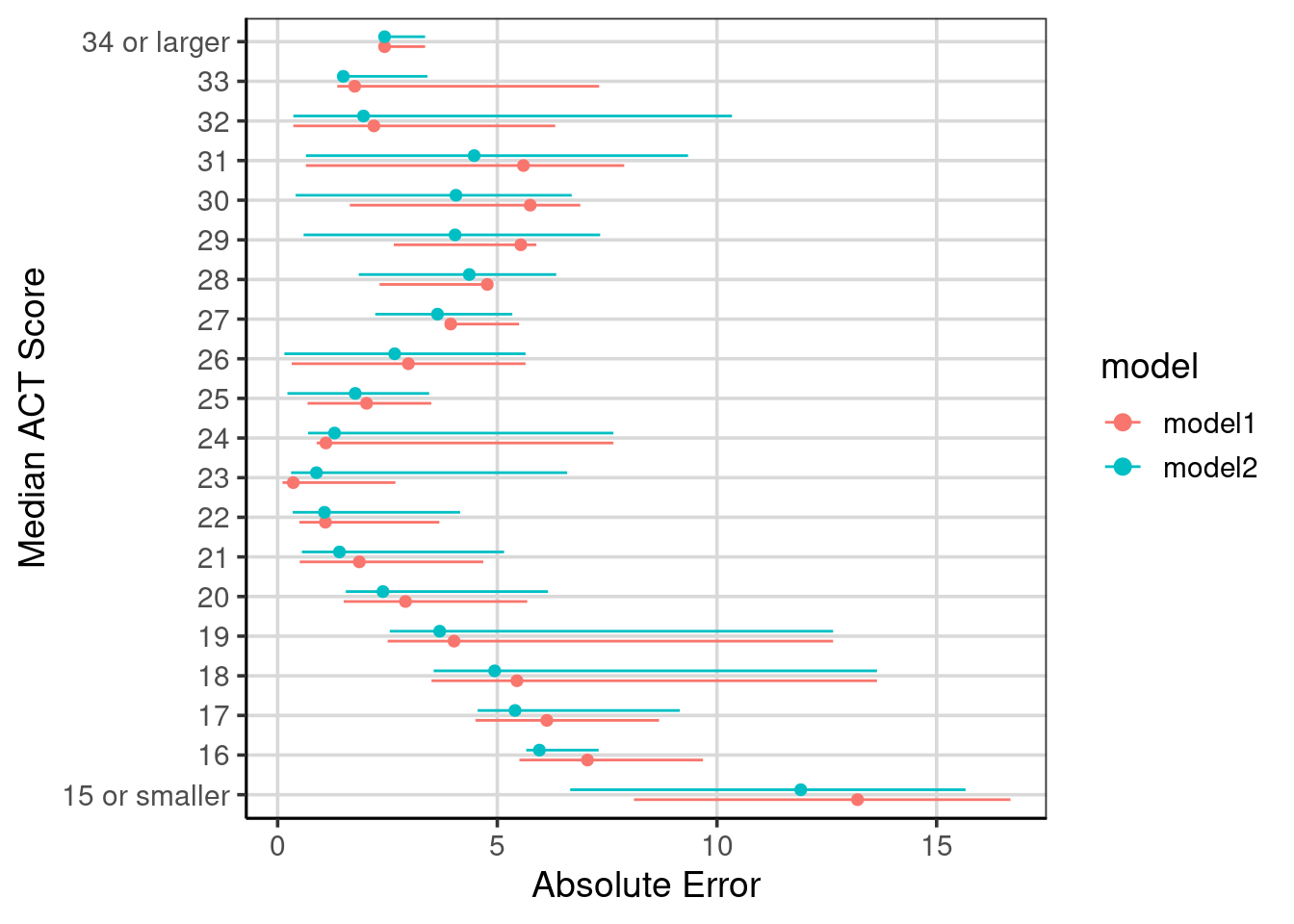Figure 6.9: Compared absolute error across the two regression tree models fitted.

Comparison of the conditional error can be helpful to evaluate areas of the model that improved and if any other areas did not improve or were worse.

To evaluate how well this model can do, all of the attributes will be open to be included in the final model. The only one that is removed from the main data is the institution name. This can be done with the . character after the ~ in the model formula. The model tree is not printed as it is large and not able to be easily viewed. Instead, the evaluation of accuracy will be compared to the other two models fitted. The attributes included in the final model though can be accessed and ranked by those that are the most important.

act_reg_sat <- rpart(actcmmid ~ .,
data = select(colleges, -instnm),
method = "anova")

colleges_pred <- colleges_pred %>%
mutate(act_pred_sat = predict(act_reg_sat),
error_sat = actcmmid - act_pred_sat)

conditional_error_sat <- colleges_pred %>%
df_stats(abs(error_sat) ~ act_recode, mean, median, min, max, length)

act_reg_sat\$variable.importance
##           city tuitionfee_out  tuitionfee_in       costt4_a       adm_rate
##    10608.27504     8438.13169     7869.78358     6700.46334     2858.66475
##         stabbr       debt_mdn  grad_debt_mdn           ugds         region
##     1420.67466      696.36673      570.77579      210.91393       76.49506
##         locale
##       53.73178

The table below shows the attributes that are the most important, with those at the top of the table being more important than those at the bottom of the table. Notice that the most important seems to be the city, followed by the tuition, then cost, and so on.

x
city 10608.27504
tuitionfee_out 8438.13169
tuitionfee_in 7869.78358
costt4_a 6700.46334
stabbr 1420.67466
debt_mdn 696.36673
ugds 210.91393
region 76.49506
locale 53.73178

The evaluation accuracy of this model that could contain most of the attributes in the data is shown in Figure 6.10. The figure also shows the comparisons to the other two models explore in this chapter, labeled as ‘model1’ and ‘model2’ respectively. Notice the improvement across all of the median ACT scores for the model that was fitted with the ability to include all of the attributes.

bind_rows(
mutate(conditional_error, model = 'model1'),
mutate(conditional_error2, model = 'model2'),
mutate(conditional_error_sat, model = 'model_sat')
) %>%
mutate(model = factor(model, levels = c('model1', 'model2', 'model_sat'))) %>%
gf_pointrangeh(act_recode ~ mean + min + max,
color = ~ model,
position = position_dodge(width = 0.5)) %>%
gf_labs(y = "Median ACT Score",
x = "Absolute Error")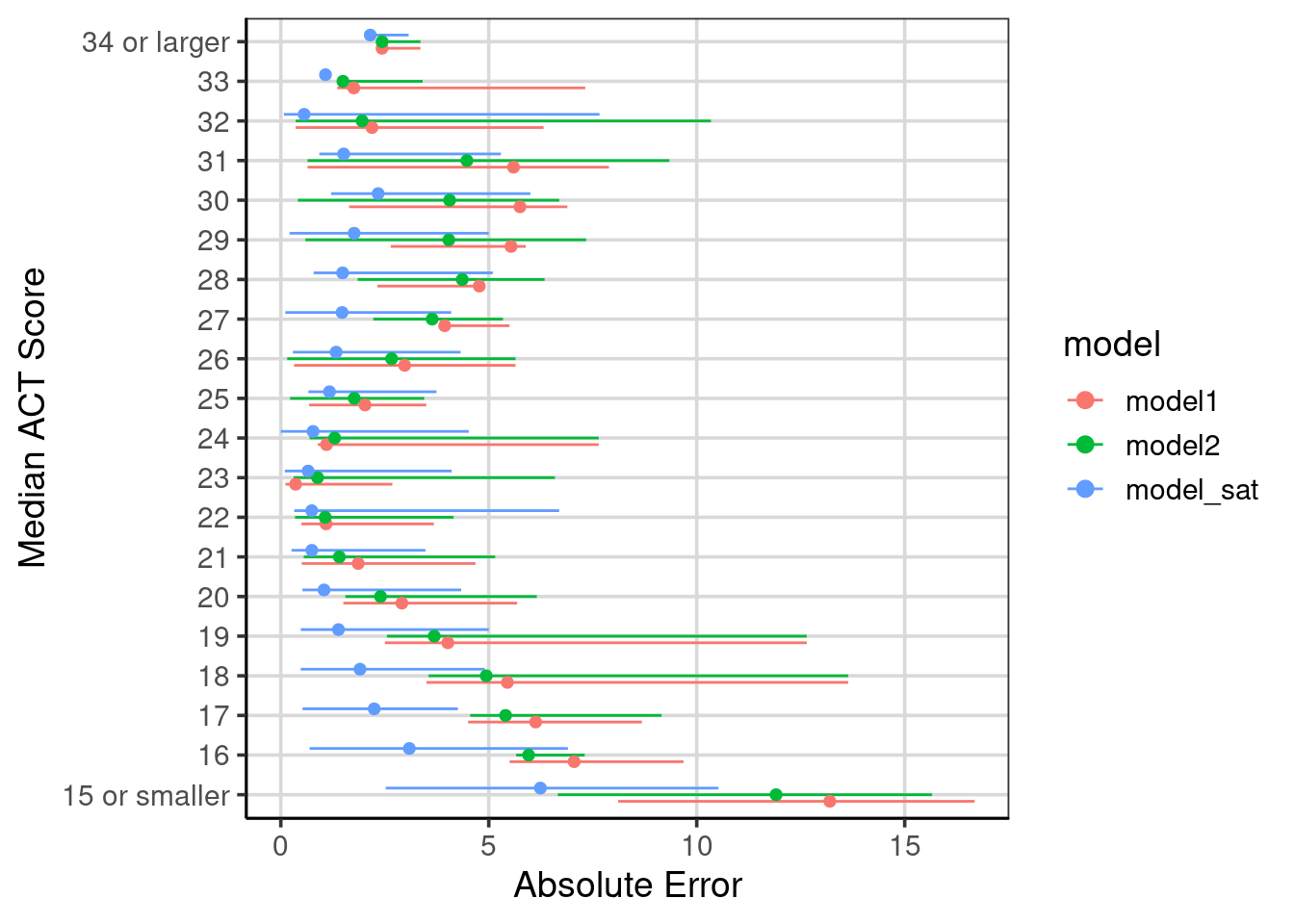Figure 6.10: Evaluation of model accuracy across the three models fitted.